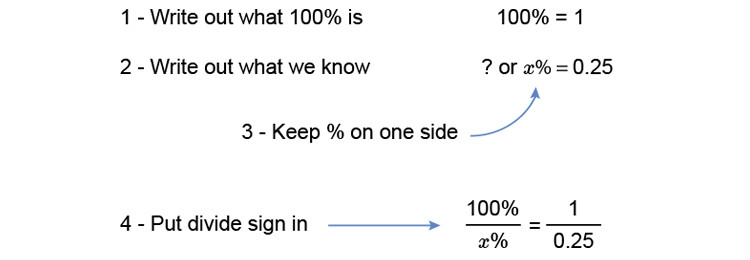# Decimals to percentages

1. Change 0.25 to a percentageMultiply both sides by x to get x on its own. (What you do to one side do to the other).

(100timescancelx)/cancelx=(1timesx)/0.25

100=x/0.25

Multiply both sides by 0.25 to get x on its own.

0.25times100=(xtimescancel0.25)/cancel0.25

x=100times0.25

x=25

Answer: x=25%